• 写在前头：说到数字图像处理，不得不提起MATLAB。这是一款非常方便的仿真软件，绝大多数的图像处理可以用MATLAB完成。 　有人问，处理图片，用PS岂不是更好。两者各有优点，如果需要将1００００幅图片转换成灰度...
写在前头：说到数字图像处理，不得不提起MATLAB。这是一款非常方便的仿真软件，绝大多数的图像处理可以用MATLAB完成。
有人问，处理图片，用PS岂不是更好。两者各有优点，如果需要将1００００幅图片转换成灰度图像并保存呢？MATLAB只需要一段很短的程序运行几秒就可以完成这个工作。
本文基于MatlabR2012a，将由浅入深写下去。

MATLAB中图像的基本操作

1、读取、显示图片

imread：
A = imread(filename, fmt)

A是结构体名，用来存储读入的图像数据。filename是读取的文件名，文件名要用”括起来。fmt是读取文件的类型如：jpg、png等等，这个参数可以不输入，由MATLAB自动判断。
显示函数imshow()原型：

    imshow：
imshow(I)

I为读取后保存在MATLAB中的结构体名。
程序实例：

A=imread('1.jpg');％读取名为１．ｊｐｇ的图片
imshow(A)％显示图片２、将灰度图片变成负片

对图像进行操作，实际上是将图像看成许多个像素点，对每个像素点进行操作。在计算机系统中，灰度图片被看成是许多个由值在［０～２５５］之间的像素点组成的图像，２５５表示白色，０表示黑色，黑白之间存在２５６个灰度级。负片是指将原灰度图白色的地方变成黑色，黑色的地方变成白色。也就是将０变成２５５，２５５变成０。ＭＡＬＴＡＢ的ｉｍａｄｊｕｓｔ（）函数提供了该功能。其函数原型：

imadjust：
J = imadjust(I,[low_in; high_in],[low_out; high_out])

其中，Ｉ为原灰度图像，ｌｏｗ＿ｉｎ，ｈｉｇｈ＿ｉｎ为输入图像的低和高灰度级，设置为［０，１］表示从０～２５５的归一化，ｌｏｗ＿ｏｕｔ，ｈｉｇｈ＿ｏｕｔ为输出图像的低高灰度级。
若是想将图片转换为负片，那么将[low_in; high_in]设置为［０，１］，将[low_out; high_out]设置为［１，０］。即原来输入为０的地方变成１输出，输入为１的地方变成０输出。
实例：

　A=imread('1.jpg');
imshow(A)％显示原图
figure,imshow(A1)％显示负片３、彩色图片转换成灰度图片

我们在计算机中看到的大多数彩色图片是基于ＲＢＧ三通道的图片，红绿蓝三种颜色，每一种取值均为［０～２５５］。通过２５５＊２５５＊２５５，可以构成庞大的颜色群。而灰度图像只有２５６个灰度级。通过以下公式可以将ＲＧＢ转换成灰度
ＧＲＡＹ＝0.2989  R + 0.5870 * G + 0.1140 * B*
ＭＡＴＬＡＢ中提供的函数ｒｇｂ２ｇｒａｙ为我们提供了将彩色图片转换成灰度图片的功能。函数原型：

I = rgb2gray(RGB)

实例：

 A=imread('001.png');％原彩色图片
imshow(A)
A_gray=rgb2gray(A);％转换成灰度图片
figure,imshow(A_gray)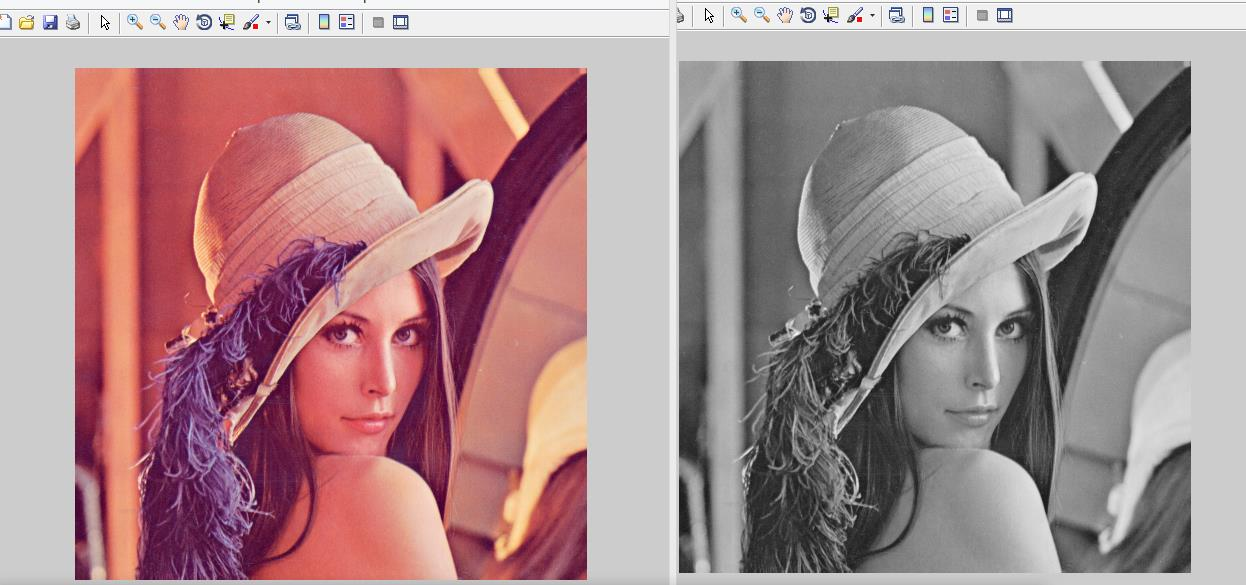展开全文图像处理 matlab 图片
• 一、图像处理预备知识1、图像处理(image processing)用计算机对图像进行分析，以达到所需结果的技术。又称影像处理。图像处理一般指数字图像处理。在计算机中，按照颜色和灰度的多少可以将图像分为二值图像、灰度...
一、图像处理预备知识

1、图像处理(image processing)

用计算机对图像进行分析，以达到所需结果的技术。又称影像处理。图像处理一般指数字图像处理。

在计算机中，按照颜色和灰度的多少可以将图像分为二值图像、灰度图像、索引图像和真彩色RGB图像四种基本类型。

2、图像处理常用方法

1）图像变换

为了用正交函数或正交矩阵表示图像而对原图像所作的二维线性可逆变换。一般称原始图像为空间域图像，称变换后的图像为转换域图像，转换域图像可反变换为空间域图像。

它可以分为四个部分：空域变换等维度算法，空域变换变维度算法，值域变换等维度算法和值域变换变维度算法。其中空域变换主要指图像在几何上的变换，而值域变换主要指图像在像素值上的变换。等维度变换是在相同的维度空间中，而变维度变换是在不同的维度空间中，例如二维到三维，灰度空间到彩色空间。

常用的有三种变换方法：傅里叶变换、沃尔什-阿达玛变换、离散卡夫纳－勒维变换。这里只讲傅里叶变换。

2）图像增强和复原

图像增强不增加图片信息，只对感兴趣的特征进行强调突出，增加辨别能力。根据人的主观评定，具有一定的人为畸变。一般通过空间域处理和频率域处理两种方法。

图像复原技术的主要目的是以预先确定的目标来改善因散焦、像物相对运动、成像器材的图固有缺陷和外部干扰导致退化的图像。

图像增强和复原的目的是为了提高图像的质量，如去除噪声，提高图像的清晰度等。图像增强主要是一个主观过程，而图像复原则大部分是一个客观的过程。

3）图像压缩

图像压缩是一种减少描绘一幅图像所需数据量的技术和科学，它是数字图像处理领域中最有用和商业上最成功的技术之一。

图像压缩既可以是无损压缩，没有信息损失；也可以是有损压缩，不能通过解压恢复原图，牺牲准确度换来压缩能力的增加。

4）图像分割

图像分割是数字图像处理中的关键技术之一。分割将图像细分为构成它的子区域或物体。细分程度取决于要解决的问题。即将图像中有意义的特征部分提取出来，其有意义的特征有图像中的边缘、区域等，这是进一步进行图像识别、分析和理解的基础。

5）图像描述

图像描述是图像识别和理解的必要前提。作为最简单的二值图像可采用其几何特性描述物体的特性，一般图像的描述方法采用二维形状描述，它有边界描述和区域描述两类方法。

6）图像识别

图像经过某些预处理（增强、复原、压缩）后，进行图像分割和特征提取，从而进行判决分类。

二、MATLAB简介

Matlab是一个高级的矩阵/阵列语言，它包含控制语句、函数、数据结构、输入和输出和面向对象编程特点。用户可以在命令窗口中将输入语句与执行命令同步，也可以先编写好一个较大的复杂的应用程序（M文件）后再一起运行。

三、MATLAB图像处理代码

1、图像读取及保存

MATLAB的基本数据单位是矩阵，一幅图像可定义为一个二维函数f(x,y)，其中x和y是空间（平面）坐标，而在任何一对空间坐标(x,y)处的幅值f称为图像在该点处的强度或灰度。当x，y和灰度值f是有限的离散数值时，我们称该图像为数字图像。在MATLAB中，数字图像就是一个个矩阵。

1）图像读取

举例：

2）图像保存

函数：imwrite(OutImg, ‘图像文件’)：输出内存中图像数据到文件

举例：

imwrite(OutImg,'d:\photo\yn_ln.jpg'); %将OutImg矩阵表示的图像存储到电脑D盘photo文件夹中，并命名为yn_ln的.jpg格式的图像。

3）图像显示

函数：imshow(ImgData) ：将图像文件显示到屏幕
subplot(行数，列数，区域索引);

举例：

subplot(1,1,1);imshow(InImg); %将InImg图像文件显示到屏幕上指定位置

4）图像转换

I=rgb2gray(rgbImg)： 将彩色图像转换为灰度图像，rgbImg为彩色图像bw=im2bw(Img,level)： 将灰度图像转换为二值图像，Img为灰度图像，level为0~1的灰度级阈值；原图灰度图像                                                         二值图像

2、图像增强

1）数字图像灰度增强

J=imadjust(I,[low high], [bottom top],gamma): 对灰度图像进行直接灰度变换；I为增强前灰度图像，J为增强后的灰度图像，[low high]增强前灰度级范围，[bottom top]增强后灰度级范围

举例：

clear;
I=rgb2gray(InImg);
bw1=im2bw(I,0.8);
bw2=im2bw(J,0.8);
bw2=medfilt2(bw2);
subplot(1,2,1);imshow(bw1);
subplot(1,2,2);imshow(bw2);增强前                                                                                              增强后

2）数字图像空域滤波

S=medfilt2(J): 对图像进行中值滤波
举例：

M=0;V=0.01;
J1=imnoise(I, 'gaussian',M,V);
S=medfilt2(J1);
subplot(1, 2, 1); imshow(J1);
subplot(1, 2, 2); imshow(S);高斯噪声                                                                                        中值滤波

3）数字图像频域滤波

F=fft2(I): 2D 傅里叶正变换

Pha=angle(F): 计算相位谱

R= abs(F): 计算功率谱F1=real(F): 获得傅里叶变换实部分量F2=imag(F): 获得傅里叶变换虚部分量fftshift(F): 移动傅里叶频谱中心至零频率

I=ifft2(F): 2D 傅里叶逆变换

举例：（1）

I=BWBand(21,31);
F=fft2(I);                        %傅里叶正变换
F=fftshift(F);
L=abs(F);
L= mat2gray(L);
I3=imrotate(BWBand(21, 31),30);
F3=fft2(I3);
F3=fftshift(F3);
L3=abs(F3);
L3= mat2gray(L3);
subplot(141); imshow(I);
title('原图');
subplot(142); imshow(I3);
title('旋转30度图');
subplot(143); imshow(L);
title('功率谱图');
subplot(144); imshow(L3);

title('旋转30度功率谱图');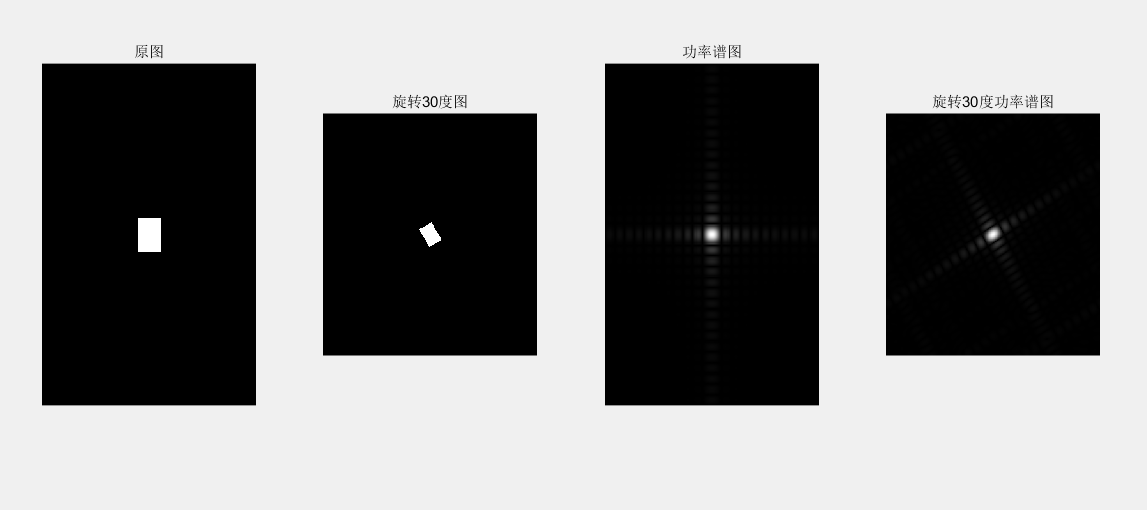subplot(2, 2, 1); imshow(InImg);
title('原彩色图');
I=rgb2gray(InImg);
J1=imnoise(I, 'salt & pepper',0.01);
subplot(2, 2, 2); imshow(J1);
title('椒盐噪声污染图');
[f1, f2]=freqspace([199 200],'meshgrid');        %产生等间隔采样的频率响应
R=sqrt(f1.^2+ f2.^2);                   %计算功率谱
Hd=ones([199 200]);
Hd(R>0.5)=0;                                   %产生理想的带通滤波器，频率带宽[0.1 0.5]
win= fspecial('gaussian',[199 200],2); %采用高斯函数生成同样大小的窗函数
win= win./max(win(:));                       %窗口函数归一化
H=fwind2(Hd,win);                           %利用窗口函数产生实际的带通滤波器
subplot(2, 2, 3); freqz2(H);
ff= imfilter (J1,H);
subplot(2, 2, 4); imshow(ff);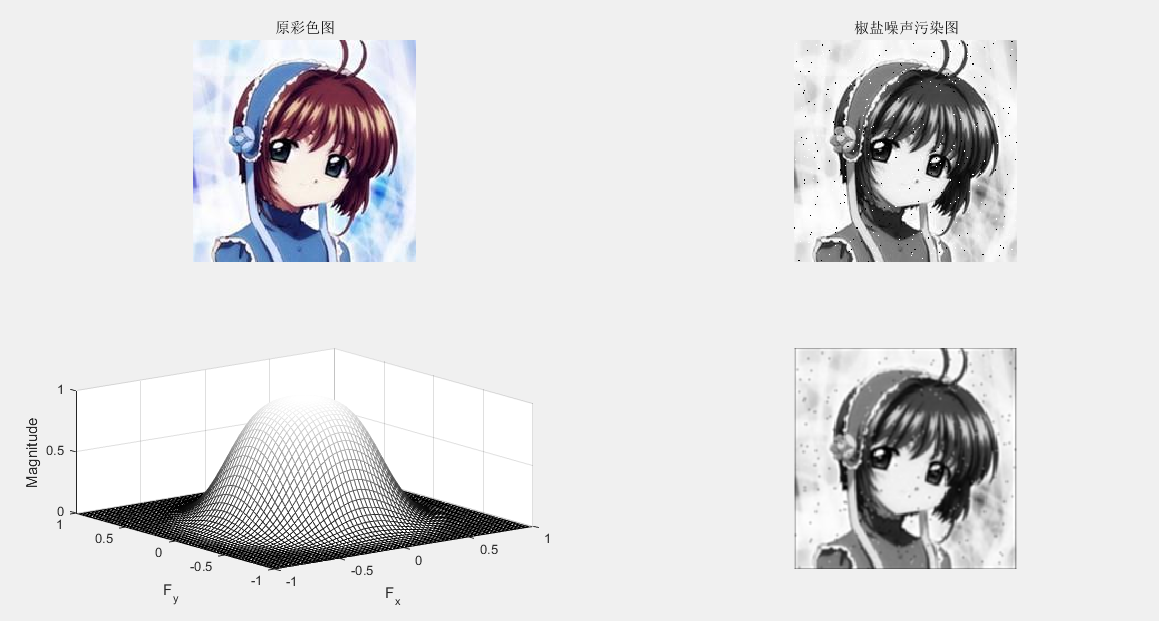3、图像复原

图像滤波：
J = WIENER2(I,[M N]): 对灰度图像实施维纳自适应滤波 ，[M N]像素领域大小C=conv2(A,B) : 计算矩阵 A 和 B 的卷积

举例：

I=rgb2gray(InImg);
J1=imnoise(I, 'gaussian');
B1=[0 0.2 0;0.2 0.2 0.2; 0 0.2 0];   %平滑滤波器
H = fspecial('average',[5 5]);
Blurred1 = imfilter (J1,H);
H = fspecial('gaussian',[5 5]);
Blurred2 = imfilter (J1,H);
K = wiener2(J1,[5 5]);
subplot(1, 5, 1); imshow(J1) ; %显示噪声图像
title('高斯噪声图像');
subplot(1, 5, 2); imshow(Blurred1) ; %显示均值滤波图像
title('均值滤波图像');
subplot(1, 5, 3); imshow(Blurred2) ; % 显示高斯滤波图像
title('高斯滤波图像');
subplot(1, 5, 4); imshow(K);% 显示维纳滤波器滤波图像
title('维纳滤波器滤波图像');
C1=conv2(J1,B1, 'same'); %对加噪后的图像平滑滤波
C1=mat2gray(C1); %将矩阵转换为灰度图像
subplot(1, 5, 5); imshow(C1);% 显示平滑图像
title('平滑图像');4、图像压缩

哈夫曼编码：无损压缩

步骤：1）缩减信源 2）尽可能少的调动信源符号排序 3）规定大概率事件编码

clear
close all;  %定义HufData/Len为全局变量的结构体
global HufData;
global Len;
disp('计算机正在准备输出霍夫曼编码结果,请耐心等待……'); %原始码字的灰度
a=rgb2gray(a);
figure;
subplot(1,2,1); imshow(a);
%取消坐标轴和边框
axis off
box off
title('Tokyo Towers','fontsize',13);
subplot(1,2,2);
axis off
box off
imhist(a);
title('图像灰度直方图','fontsize',13);%图像的灰度统计
GrayStatistics=imhist(a);
GrayStatistics=GrayStatistics';
GrayRatioo=GrayStatistics/sum(GrayStatistics);
GrayRatioNO=find(GrayRatioo~=0);
GrayRatioyes=find(GrayRatioo==0);
Len=length(GrayRatioNO);  %初始化灰度集，防止系统随即赋予其垃圾值
GrayRatio=ones(1,Len);
for i=1:Len
GrayRatio(i)=GrayRatioo(i);
end
GrayRatio=abs(sort(-GrayRatio));
for i=1:Len
HufData(i).value=GrayRatio(i); %将图像灰度概率赋予结构体
end  % 霍夫曼编码/霍夫曼编码
HuffmanCode(Len); %输出码字
lavg1=0;
for i=1:Len
tmpData=HufData(i).code
str='';
for j=1:length(tmpData) ;
str=strcat(str,num2str(tmpData(j)));
disp(strcat('a','= ',str));
end
len1=length(tmpData) ;
lavg1=lavg1+GrayRatio(1,i)*len1;

end
ratio_delet=8/lavg1;
str='';
str=strcat(str,num2str(ratio_delet));

disp(strcat('压缩率','= ',str))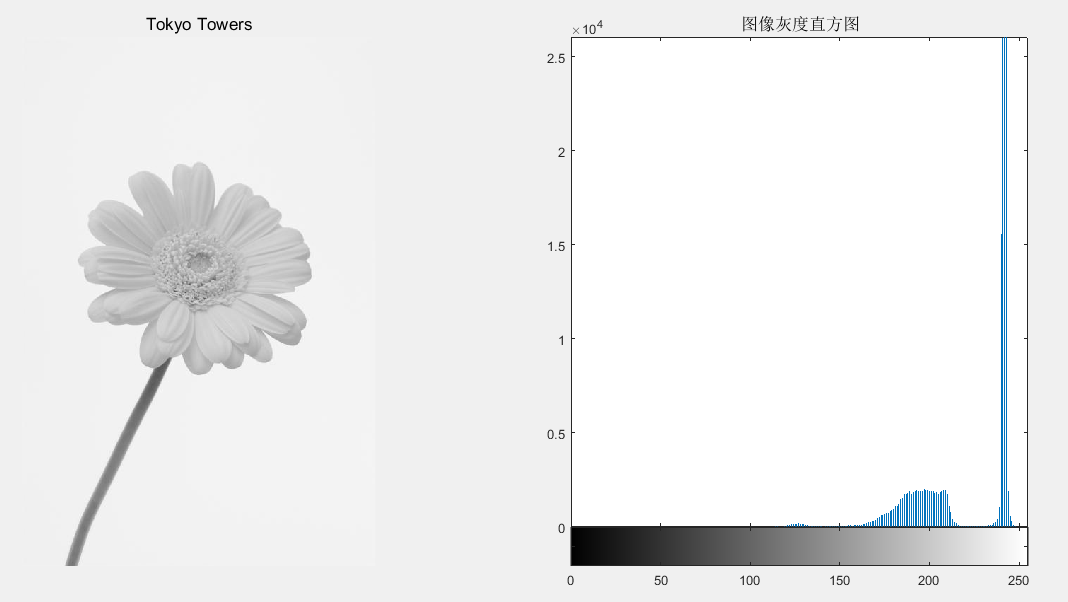5、图像分割（边缘提取）

1）图像几何纠正、边缘检测

B=imresize(A,m,type): 图像大小调整

B=imrotate(A,angle,method): 图像旋转调整

最近邻插值法：将变换后的图像中的原像素点最邻近像素的灰度值赋给原像素点的方法
双线性插值法：核心思想是在两个方向分别进行一次线性插值。

举例：

I=rgb2gray(InImg);
B1=imresize(I,1.5, 'nearest');
B2=imresize(I,1.5, 'bilinear');
C1= imrotate (I,45, 'nearest');
C2= imrotate (I,45, 'bilinear');
subplot(231);imshow(I) ;%显示原始图像于
title('原始图像');
subplot(232); imshow(B1) ;%显示调整图像(最近邻插值)
title('最近邻插值图像');
subplot(233); imshow(B2) ;% 显示调整图像(双线性插值)
title('双线性插值图像');
subplot(234); imshow(C1);% 显示旋转图像(最近邻插值)
title('旋转最近邻插值图像');
subplot(235); imshow(C2);% 显示旋转图像(双线性插值)
title('旋转双线性插值');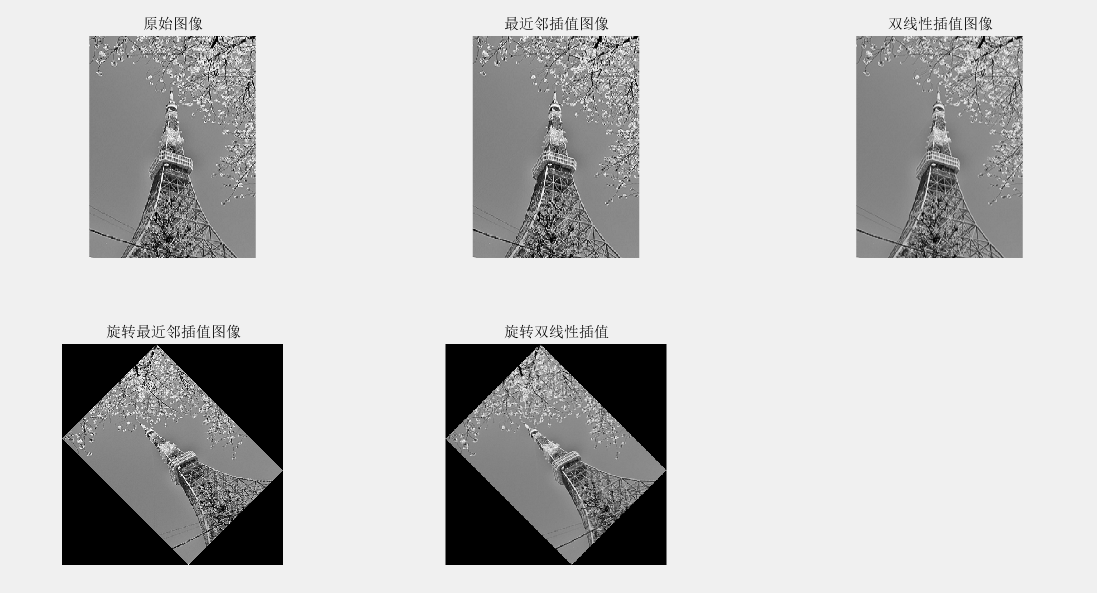2）边缘提取

BW=edge(I,method,thresh): 检测灰度图像的边缘

举例：

I=rgb2gray(InImg);
BW1= edge (I, 'sobel',0.1);%sobel边缘检测器
BW2= edge (I, 'prewitt',0.1);%prewitt边缘检测器
BW3= edge (I, 'roberts',0.1);%roberts边缘检测器
BW4= edge (I, 'log',0.01,2.0);%log边缘检测器
subplot(221); imshow(BW1) ;% 显示边缘图像
title('sobel边缘图像');
subplot(222); imshow(BW2) ;%显示边缘图像
title('prewitt边缘图像');
subplot(223); imshow(BW3) ;% 显示边缘图像
title('roberts边缘图像');
subplot(224); imshow(BW4) ;% 显示边缘图像
title('log边缘图像');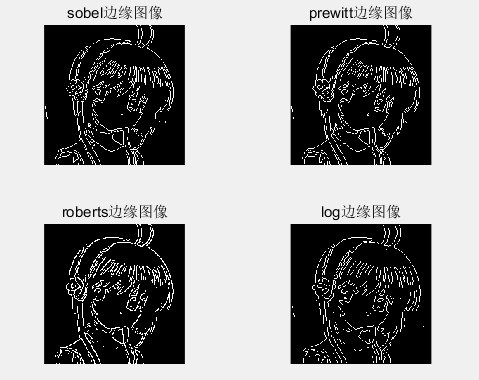边缘提取


展开全文• 一、imfinfo函数——查看图像文件信息，注意参数是文件路径和文件名，不是图像对应的矩阵。 &gt;&gt; imfinfo('C:\Users\zhulf\Desktop\1.jpg') ans =   Filename: 'C:\Users\zhulf\Desktop\1.jpg'  ...
一、imfinfo函数——查看图像文件信息，注意参数是文件路径和文件名，不是图像对应的矩阵。

>> imfinfo('C:\Users\zhulf\Desktop\1.jpg')

ans =
Filename: 'C:\Users\zhulf\Desktop\1.jpg'
FileModDate: '19-Apr-2015 08:40:54'
FileSize: 1528413
Format: 'jpg'
FormatVersion: ''
Width: 1548
Height: 1548
BitDepth: 24
ColorType: 'truecolor'
FormatSignature: ''
NumberOfSamples: 3
CodingMethod: 'Huffman'
CodingProcess: 'Sequential'
Comment: {}
Make: 'Smartisan'
Model: 'Smartisan T1'
Orientation: 0
DateTime: '2015:04:19 08:40:53'
DigitalCamera: [1x1 struct]
GPSInfo: [1x1 struct]
MeteringMode: 'unknown'
LightSource: 'unknown'
Flash: 16

二、imshow函数

1、imshow(I)  显示图像I

Matlab代码：

>> imshow(I)

显示结果2、imshow(I,[low high])  它显示的是像素处理后的图像I，注意的是它只是显示的时候改变了图像像素，实际上并没有改变图像像素，图像像素值还是原来的值。

Matlab代码：

>> imshow(I,[0 80])它对图像像素的处理是：将I中像素值大于等于high变成high，将小于等于low的变成low，再将[low,high]中间的像素映射为默认的像素级，一般为[0,255]。

我们把上述描述转为代码：

mmax=max(I(:));

mmin=min(I(:));

for i=1:256

for j=1:256

if I2(i,j)<=0 I2(i,j)=0;

elseif I2(i,j)>=80

I2(i,j)=255;

end

end

end

I3=I2;

for i=1:256

for j=1:256

I3(i,j)=255/80*I3(i,j);

end

end

imshow(I3)

显示图像结果为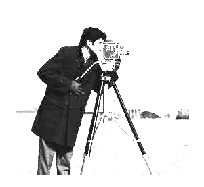和imshow(I,[0 80])效果是一样的。

3、 imshow(I,[ ])  它等同于imshow(I,[min(I:),max(I:)])，可以理解为把图像I转化成0-255灰度级来显示。

我们在这里做一个实验：下面三幅图分别为imshow(I)，imshow(I,[])和把图像像素值映射为[0,255]所显示的图像。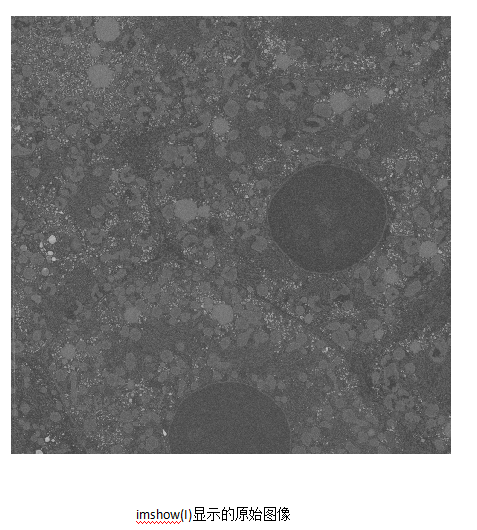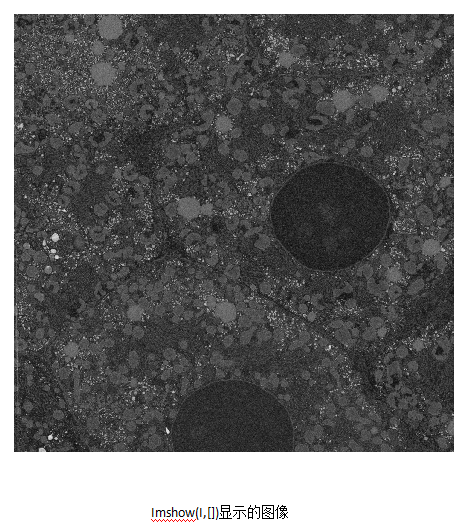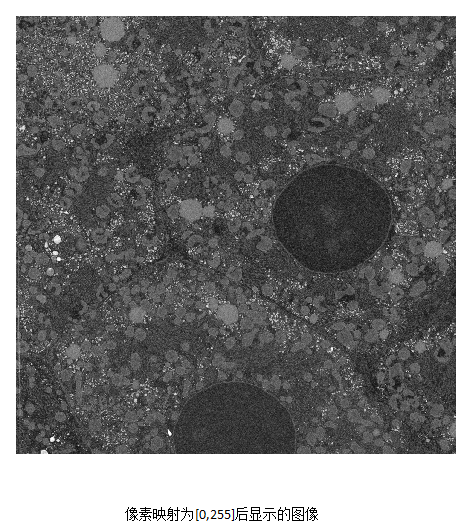我们发现，imshow(i,[])显示的效果正和把像素值映射为[0,255]效果所显示的一样。

Matlab代码如下：

mmax=max(A(:));

mmin=min(A(:));

for i=1:4096

for j=1:4096

A2(i,j)=1.0*255/(mmax-mmin)*(A(i,j)-mmin);

end

end

imshow(A)

figure,imshow(A,[])

figure,imshow(A2)

再例如，一个灰度级为17-2424的int16类型的图像，matlab显示(imshow(I))效果如下，可见，显示效果并不好，接下来我们使用imshow(I,[])，显示结果如下：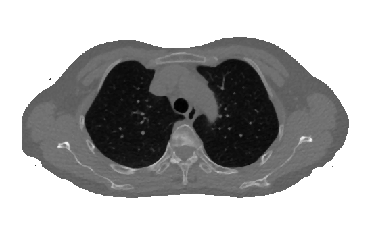显示结果较好，对比度较高。同样，imshow(I,[])的效果等同于把像素映射到0-255灰度级，映射后图像如下：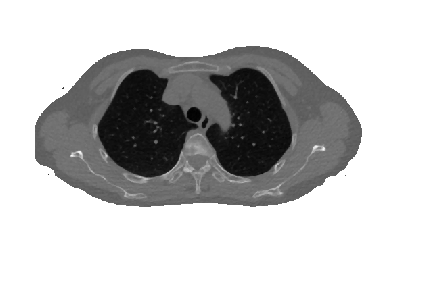实现灰度映射的Matlab如下：

mmax=double(max(A(:)));  %类型转换很重要

mmin=double(min(A(:)));  %类型转换很重要

for i=1:512

for j=1:512

tmp=double(A(i,j));  %类型转换很重要

A2(i,j)=uint8(255*(tmp-17)/2407);  %类型转换很重要

end

end

imshow(A)

figure,imshow(A,[])

figure,imshow(A2)

上面是对imshow()函数的理解，不明白的欢迎留言交流。

三、rgb2gray 将彩色图像转换为灰度图像

>> B=rgb2gray(A);
>> imshow(A)

>> figure,imshow(B)

四、数据类型转换函数

im2uint8  将图像转换为uint8类，相应的像素值也会变化到相应范围，如对uint16类图像应用im2uint8，则像素值由0-65535变化成0-255，这是一种线性映射。

im2uint16 将图像转换为uint16类，也有上面如此的性质。

im2double 将图像转化为double类，必要时缩放像素值。如果图像img是double型的，d=img;如果图像是logical或single型图像，d=double(img);如果图像是uint8型，d=double(img)/255；如果图像是uint16型，d=double(img)/65535。

im2double一般会将uint8归一化到[0,1],而double只是将数据变为double,如：

a = uint8(250);
b = double(a)
c = im2double(a)
b =
250
c =
0.9804

im2single 将图像转化为single类，性质和im2single类似。

mat2gray 可以将图像转换为标定[0,1]范围的double类的图像。

调用的语法是：

g=mat2gray(A,[Amin,Amax])，其中g具有0（黑）到1（白）的值。参数Amin使得A中小于Amin的值，在g中变为0；而在A中大于Amax的值，在g中变为1。

也可以是:

g=mat2gray(A)，

Amin,Amax默认为A中实际的最大值和最小值，它独立于输入的类，将整个输入值的范围标定为[0,1]。

>> f=[1 2;3 4]
f =
1     2
3     4
>> g=mat2gray(f)
g =
0    0.3333
0.6667    1.0000

BW = im2bw(I,LEVEL)   二值化图像，将大于LEVEL的像素值变为1，小于LEVEL的像素值变为0，且图像像素变成逻辑类；LEVEL是阈值，在[0,1]之间。

>> gb=im2bw(g,0.6)
gb =
0     0
1     1

>> gbv=islogical(gb)
gbv =
1

五、whos

B为图像矩阵

>> whos B
Name         Size                Bytes  Class    Attributes
B         1548x1548            2396304  uint8

六、反转图像

>> imshow(I)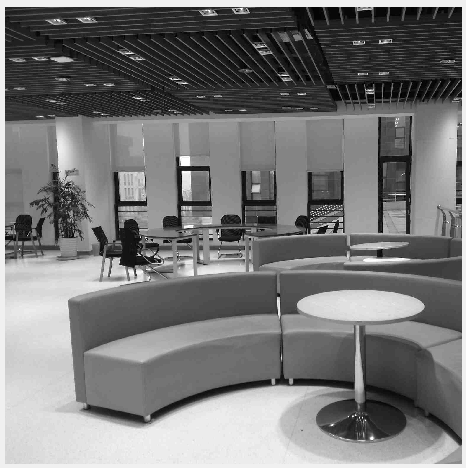上下翻转：

>>I2=I(end:-1:1,:);

>> figure,imshow(I2)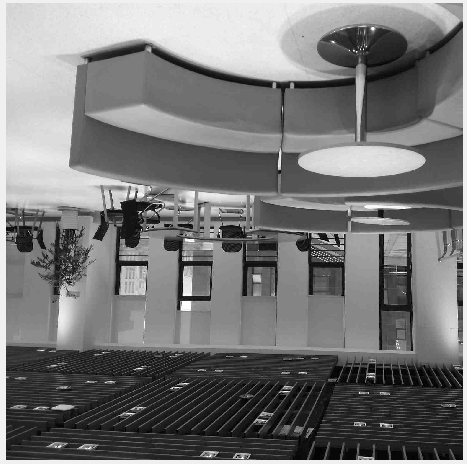上下左右均反转：

>> I3=I(end:-1:1,end:-1:1);
>> figure,imshow(I3)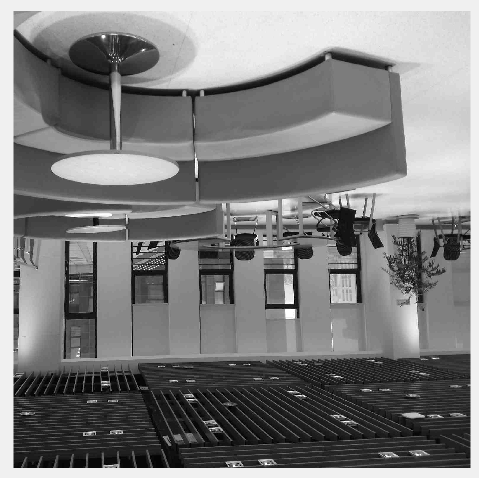七、抽取图像（压缩图像）

>> I4=I(520:855,90:837);
>> figure,imshow(I4)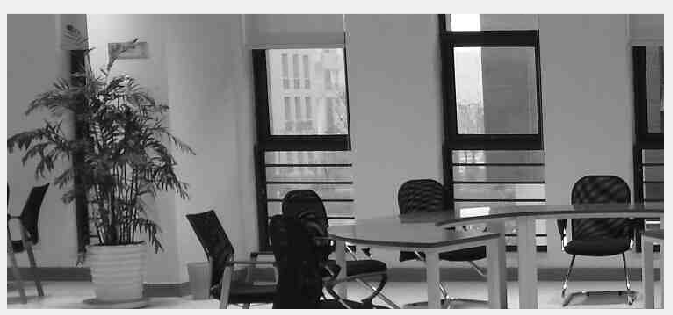图像也由原来的1548 x 1548变成了336 x 748。

>> I5=I(1:2:end,1:2:end);
>> figure,imshow(I5)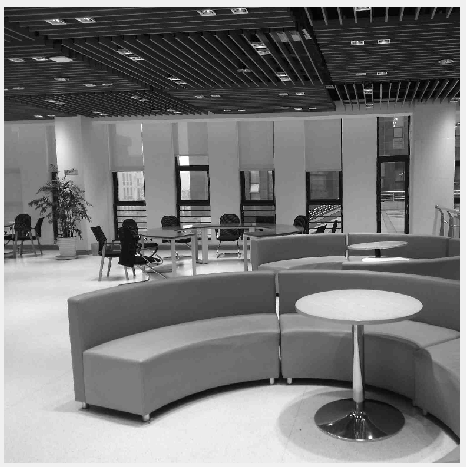图像由1548 x 1548 变成了774 x 774，filesize由99745变成了76747，做了部分压缩，但清晰度差不多。

八、improd()函数

function[p,pmax,pmin,pn]=improd(f,g)
%IMPROD Computes the produce of two images.
%[P,PMAX,PMIN,PN]=IMPROD(F,G) outputs the element-by-element
%product of two input images,F and G,the product
%maximum and minimum values,and a normalized product array with
%values in the rang[0,1].The input images must be of the same
%size.They can be of class uint8,uint16,or double. The outputs
%are of class double.
fd=double(f);
gd=double(g);
p=fd.*gd;
pmax=max(p(:));
pmin=min(p(:));
pn=mat2gray(p);

>> [p,pmax,pmin,pn]=improd(f,g)
p =
1     4
6     4
pmax =
6
pmin =
1
pn =
0    0.6000
1.0000    0.6000

之后陆续补充。。
展开全文matlab 图像处理
• GUI，即图形用户接口，是MATLAB的可视化操作功能，本文编写一个简单GUI进行图像处理。1、新建GUI文件，点击file-&gt;new-&gt;GUI如下图所示点击GUI后出现如下图界面，默认为Blank GUI (Default)选项，点击OK...
GUI，即图形用户接口，是MATLAB的可视化操作功能，本文编写一个简单GUI进行图像处理。1、新建GUI文件，点击file->new->GUI如下图所示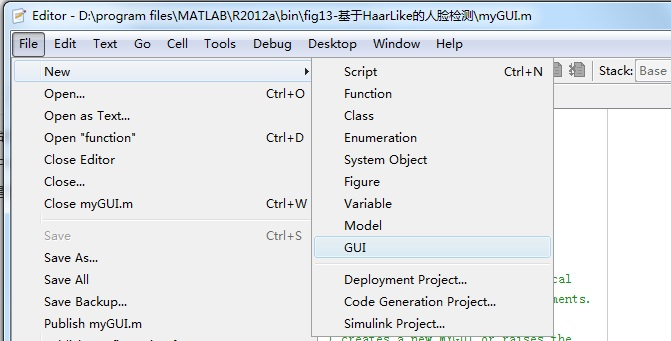点击GUI后出现如下图界面，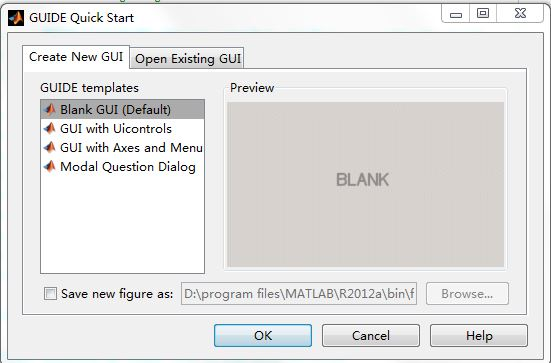默认为Blank  GUI (Default)选项，点击OK即可，出现如下图所示文件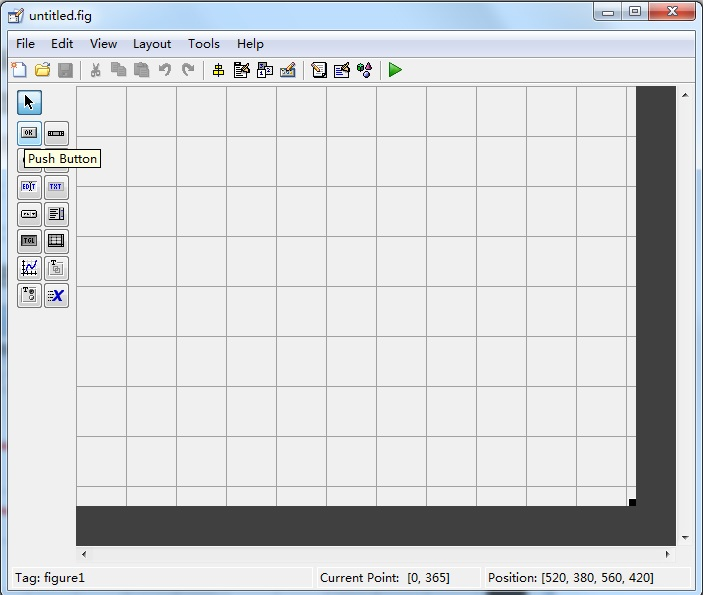将鼠标放到OK的位置会显示Push Button，为按钮，点击后在格子区域放置按钮，双击按钮，在string条目设置字符串“选择图片”，如下图所示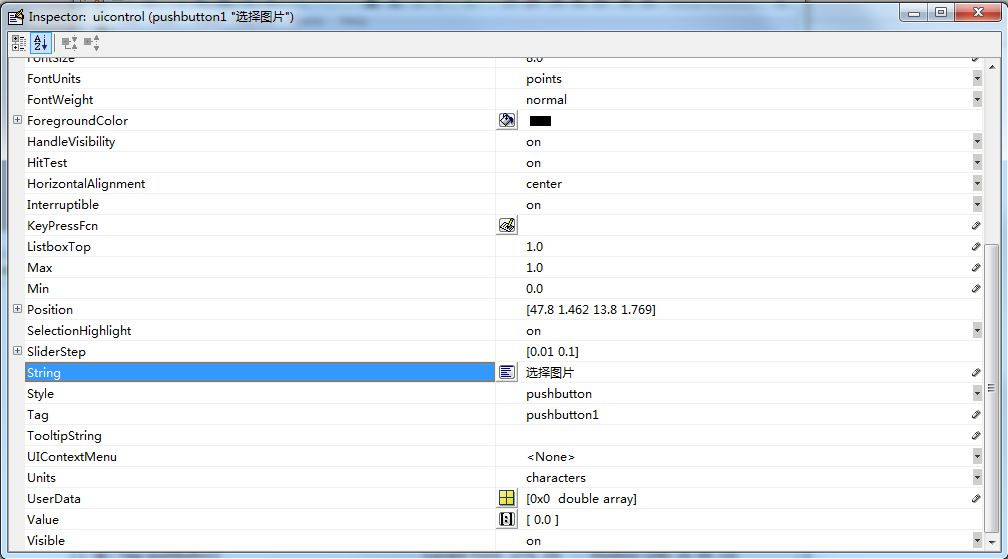然后放置可编辑文本edit和坐标系统axes，如下图所示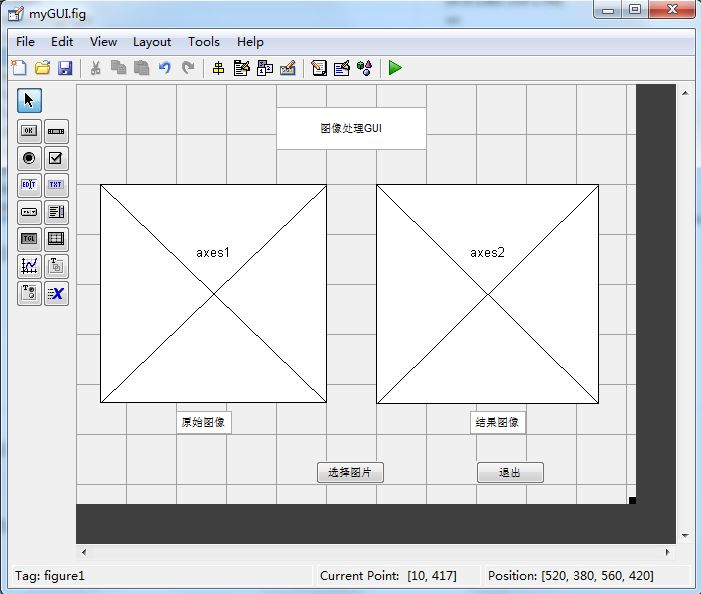保存命名为myGUI。2、编辑按钮响应事件，双击按钮，在callback条目行点击红框处，如图所示，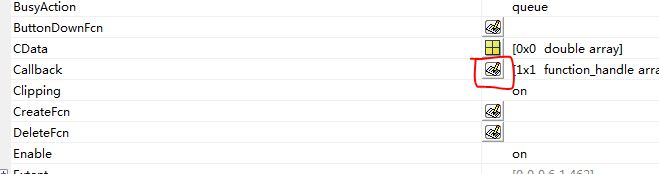在随后出现的myGUI.m文件中找到函数function pushbutton1_Callback，编写如下所示代码axis off  %%关闭坐标轴显示
[filename pathname] =uigetfile({'*.jpg';'*.bmp';'*.*'},'打开图片');

str=[pathname filename];
%%打开图像
%%打开axes1的句柄 进行axes1的操作
axes(handles.axes1);
%%在axes1中显示 图像
imshow(im);

%图像处理部分
I=im2bw(im);
BW1=edge(I,'canny'); %用canny算子进行边缘检测
path1='D:\program files\MATLAB\R2012a\bin\fig13-基于HaarLike的人脸检测\result\';
name='lena.jpg';
imwrite(BW1,[path1 name]);

str1=[path1 name];
axes(handles.axes2);
imshow(im1);  点击运行，出现如下图所示界面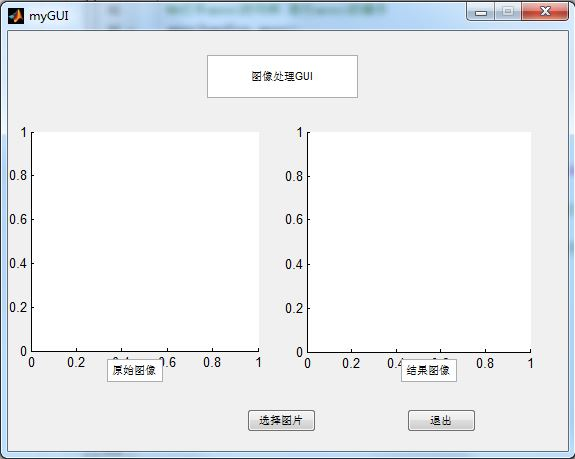在上图中点击“选择图片”，选择你想处理的图，然后在“结果图像”坐标系内显示图像处理的结果，如下图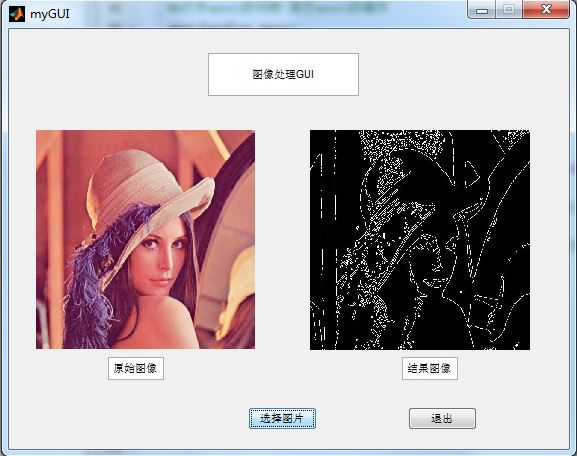展开全文MATLAB 图像处理 GUI
1，图像的读入和显示：（1）图像读入：A=imread('文件名',文件格式)
（2）图像显示：image(A);
2. 图像写回
A=imwrite('文件名',文件格式)
[X,map]=imwrite('文件名')%注意，当只写文件名时，它应带有扩展名
3.获取图像信息
info=iminfo('文件名')
4.标准图像显示技术
（1）imshow(I,n)%显示灰度图像，n为灰度级数
（2）imshow(I,[low,high])%[low,high]为图像数据的值域（可为空[ ]）
（3）imshow(BW)%显示二值图像
（4）imshow(X,map)%显示索引色图像

（4）Z=imdivide(X,y)%图像的除运算，Y也可以是常数
6.图像的类型转换（1）RGB=ind2rgb(X,map)%索引图转换为真彩色图（2）I=mat2gray(A)%将一个数据矩阵转换为灰度图（3）I=rgb2gray(RGB)%将一副灰度图转换为真彩色图（4）[X,map]=rgb2ind(RGB,n)%将RGB图转换为索引色图（5）BW=im2bw(I,level)%将真彩色图或灰度图转换为二值图，level为阈值BW=im2bw(X,map,level)%将索引色图转换为二值图
（6）I=ind2gray(X,map)%将索引色图转换为灰度图

7.傅里叶变换
（1）Y=fft2(x,m,n)%二维离散傅里叶快速变换，x为要进行傅里叶变换的矩阵，m、n是返回的变换矩阵Y的行数和列数。
（2）Y=ifft2(x,m,n)%二维离散傅里叶反变换
（3）Y1=fftshift(Y)%把傅里叶变换操作得到的结果中零频率成分移到矩阵中心，这样利于观察频谱。
8.离散余弦变换（1）D=dct2(A,m,n)%二维离散余弦变换，A是输入图像，B是返回的DCT变换系数，m、n为D的行数和列数
（2）D=idct(A,m,n)%e二维离散余弦逆变换。
（3）D=dctmtx(n)%返回DCT变换矩阵
（5）S=decorrestretch(I)%去相关色度拉伸
11.图像的增强（2）B=imfilter(A,H,option1,option2,...)A是输入图像，H是卷积核或相关核，option是一些可选参数。注：权重矩阵称为卷积核，也称为滤波器。卷积核是相关核旋转180度得到的,可选参数参看help
其中H也用H=fspecial(type,parameters)可自定义
12.中值滤波器B=medfilt2(A,[m,n])%A是输入的图像，[m,n]是邻域的大小
13.自适应滤波器
B=wiener2(A,[m,n])

14.图像的分析 P=impixel(I)%交互式获取图像像素值 P=impixel(I,c,r)%指定点坐标像素值，c、r为行坐标和列坐标
C=improfile(I,xi,yi,n,method)%创建图像强度曲线，n规定了计算图像强度点的个数，xi、yi规定了空间直线端点坐标，method是插值方法（nearest,bilinrar,bicubic）
imcontour(I,n,linespec)%显示图像数据的等值线图
15.图像的统计信息B=mean(A)%计算A的均值b=std2（A）%计算A的标准差r=corr2(A,B)%A,B为输入二维矩阵，r是返回的协方差系数

﻿﻿

展开全文• 计算机图像处理实验课1-9，看完本课程可以让你matlab技能小小进步，对图像处理稍微了解。、 （一）matlab数字图像处理实验-guide的简单使用教程 （二）matlab数字图像处理实验-图像的几何变换 （三）matlab数字...matlab 图像处理
• 经过上一篇文章的《matlab GUI图像图像基础》，我们可以知道说是入门了GUI，对于GUI图像图形还可以调用函数形成，但是对于学习图像处理来说，感觉没有那个必要去学，会了基础，就可以自己GUI图像处理功能了，主要...图像处理 GUI
• 准备学习做图像处理，首先要对图像处理里的一些基本概念有一定的了解，不然不论是Verilog还是MATLAB代码实现没有任何意义，因此总结一些有关图像处理的基本知识。数字图像处理又称为计算机图像处理，它是指将图像...
• 第一章 matlab图像处理编程基础之读取和写入文件 1.优化小技巧：提前分配矩阵内存 如memo=zeros(1024,128),这条语句用于构造一个元素全为零的矩阵，但同时也很自然地分配一块足够大的空间。 2.M文件的不能命名为...图像处理 matlab
• 1.基本函数: iread('文件路径'); idisp(); 2.一元操作： imd=idouble(im);... //将彩色图像转变成灰度图像。 ihist（）；//显示每一个像素值出现的次数 im=igamma(im,1/0.45); //伽马编码，一种非线性一...
• 整理电脑时，发现了本科时的一个matlab GUI，关于图像处理的一些基本操作，里面有挺多功能的，看下面的图片。 图像类型转化，几何运算，图像变换，添加噪声，图像复原，图像增强，图像分割，形态学处理，小波变换...图像处理 matlab gui 图像分割
• 由于这段时间在一个图像处理与三维重建的项目，其中数字图像处理的知识是必不可少的，学习matlab也有一段时间了，所以现在抽点时间将这段时间所学的东西个小总结，加深理解的同时也方便以后有需要时可以查看一下...图像处理 matlab
• MATLAB具有丰富的图像处理函数库，运算速度慢，特别是在多重循环的情况下，不适合直接应用于工程当中。如果能把MATLAB和另一种适合工程的编程语言结合到一起运用到数字图像处理领域，则会更加方便的进行图像处理，...
• 原文地址：Matlab图像处理函数汇总 作者：mimi 图像的变换  ① fft2：fft2函数用于数字图像的二维傅立叶变换，如：i=imread('104_8.tif'); j=fft2(i); ②ifft2:：ifft2函数用于数字图像的二维傅立叶反变换，如...图像处理 matlab 编程 数据 函数
• matlab数字图像处理实验 1、什么是平滑处理？  首先明白几个名词：  (1)噪声：图像噪声是指存在于图像数据中的不必要的或多余的干扰信息。 1.高斯噪声：高斯噪声是指它的概率密度函数服从高斯分布（即正态...matlab 滤波 图像处理
• 标准图像显示技术特殊图象显示技术 图像运算 代数运算空间变换图像配准 图像数据变换 二维傅立叶 离散余弦 其他变换  1）radon变换： 2）fanbeam变换：   图像分析与增强 像素值及统计 1） 2）improf
•matlab GUI 数字图像处理
• 今天发现了matlab图像处理工具箱的强大之处， 任务缘由： 需要对图像进行批处理改变尺寸 一般遇到这种任务，我们往往都会写matlab代码进行批量操作 但是matlab有自带的很强大的图像处理工具箱，  图片批处理...matlab图像处理
• 今天给matlab处理过的图像加投影信息出错，记录一下，防止以后出错，也和小伙伴们一起学习！ %% 遥感影像的读写操作（带有空间坐标信息） clc clear%读取没有空间坐标的影像 Y=imread('此处为没有投影信息的图像的...matlab图像处理
...